﻿ Empirical Correlation for an Ammonia Charged Aluminum Closed Loop Pulsating Heat PipePublications are Open
Access in this journal
Article Versions
Export Article
• Normal Style
• MLA Style
• APA Style
• Chicago Style
Review Article
Open Access Peer-reviewed

### Empirical Correlation for an Ammonia Charged Aluminum Closed Loop Pulsating Heat Pipe

Md Shahidul Haque, Antara Majumdar, Md. Faisal Kader , Md. Mahbubur Razzaq
American Journal of Mechanical Engineering. 2020, 8(1), 9-16. DOI: 10.12691/ajme-8-1-2
Received March 08, 2020; Revised April 17, 2020; Accepted May 05, 2020

### Abstract

Pulsating Heat Pipe (PHP) has become one of the promising options for passive heat transfer. This technique is especially efficient for cooling of micro and nano sized particles. This paper works on a aluminum made and ammonia charged pulsating heat pipe. The heat pipe consists of aluminum tubing having total 14 turns of 3mm and 4mm as inner and outer diameter. A constant electric power supply of 36 W was provided to run the pulsation mechanism. The experiment was carried out for three filling ratios of 0.4, 0.6 and 0.8 as well as inclination angle of 30°, 45°, 60°, 90° and 180°. The objective of this research is to formulate an empirical correlation between overall heat transfer coefficient and different physical parameter such as heat input, evaporator temperature rise, fill ratios and inclinations. It has been found that the correlation is effective for heat input and filling ratios. Experimental results showed that for these two parameters the correlation converges well.

### 1. Introduction

Closed loop pulsating heat pipe (CLPHP) is a two phase passive heat transfer device that works on continuous forming of liquid slugs and vapor plugs. The device consists of evaporator, condenser and adiabatic section. Self-excitement is created due to temperature difference between evaporator and condenser zone that circulates the annular flow throughout the tubing.

The idea of such kind of passive heat transfer device was first patented by Tesla 1 in the year 1920. Gaugler 2 developed the idea to build a heat pipe later in the year 1942. After that, industrially suitable closed loop pulsating heat pipe had been introduced by Akachi 3 in 1990. Investigations to enhance the performance of pulsating heat pipe had been carried out by changing geometrical shapes, inserting wicks, check valves, working fluid etc 4, 5, 6, 7, 8, 9, 10. Researches for the development of mathematical modeling had been carried out to predict the two phase phenomenon of pulsating heat pipe 11, 12, 13, 14. In this research work, linear empirical correlation has been tried to develop using physical parameters for the designated setup and working fluid.

### 2. Experimental Setup and Procedure

A closed loop pulsating heat pipe (CLPHP) (inside volume of 6.264×10-5m3) was made by using aluminum tube of 4.0 mm Outer Diameter (OD), 3.0 mm Inner Diameter (ID) and 8.862 m length to form 14 parallel channels with 13 bends. The CLPHP was made with 3 sections: evaporator, adiabatic and condenser section. The evaporator section is about 210 mm long, the adiabatic section is 120 mm long and the condenser section is 324 mm long. The evaporator section of the heat pipe was placed inside aluminum block (0.35m×0.21m×0.035m) having grooves of same dimension of the heat pipe to avoid any gap between the block and the pipe outside surface to ensure smooth heat flow to the CLPHP. A heating coil was fabricated by winding nichrome wire of 0.25 mm diameter on ceramic bead at a constant interval of 1.50 mm was placed inside the slot of Aluminum block which acted as heat source. Heat was supplied to the working fluid directly from AC power supply with the help of a variac. At different locations on the setup, fourteen K-type thermocouples were attached by insulated tape to monitor the temperature during the experiment. A pressure gauge (maximum range: 300psi) was employed to measure pressure variations at different sections. The experimental setup is illustrated in Figure 1.

The apparatus was placed on a wooden stand to eliminate heat loss by conduction. One gate valve was connected to the heat pipe to remove air as well as to fill the working fluid. The stand can be rotated at different orientations. At the vertical orientation (0°), the evaporator section was below the condenser section which gets reversed for 180° inclination. For the tests under horizontal orientation (90°), all sections were on the same plane. Both evaporator and adiabatic sections were having thermal insulation (0.39m×0.35m×0.075m) using layers of glass wool, while the condenser section was open to the surrounding to get cooled by natural flow of air inside the room. Figure 2 depicts the different orientation of the whole setup.

The tests were conducted between 24°C - 28°C room temperature. At first, the vacuum was created inside the pipe through vacuum pump and the gate valve made closed. The vacuum pressure was monitored by using a vacuum gauge. Liquid ammonia was taken in a syringe which was attached to the gate valve carefully. Under vacuum pressure, ammonia was injected to the pipe taking three different fill ratios as 0.4 or 2.506×10-5m3 (40% of inside volume), 0.6 or 3.758×10-5m3 (60% of inside volume) and 0.8 or 5.011×10-5m3 (80% of inside volume) and the gate valve was closed immediately to resist air insertion.

The thermocouples were first calibrated using a standard thermometer (range: -40°C~1000°C). Temperatures were recorded in boiling water of 100°, melting ice of 0°C and some other temperatures with the standard thermometer and thermocouples. One selector switch was used to monitor the temperature of five and two different points on condenser and adiabatic sections respectively and another selector switch was used to monitor the temperature of seven different points of evaporator section. Knobs were used for selecting desired thermocouples to detect temperature reading on a digital thermometer. The temperature at different sections are monitored and recorded at every 20 minutes until the steady state condition. Below 90° inclination, the system reaches steady state after about 260 minutes while beyond this position it takes more time to reach steady state even though it is not stable. For all the tests, the rise of temperature was monitored and recorded for 260 min. The input electric energy was kept constant at 36 W through variac to the aluminum block. Various parameters were measured for different inclinations at 0°, 30°, 45°, 60°, 90° and 180°, three distinct fill ratios as 40%, 60% and 80%.

• Figure 1. Schematic diagram of the experimental setup
• Figure 2. Schematic diagram representing various CLPHP setup orientations

### 3. Data Analysis and Calculations

Electric heat input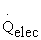is transferred to the aluminum block through nichrome wire heat coil. By conduction heat transfer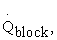heat is transferred from aluminum block to the adjacent heat pipes. After that, heat is transferred to the inside working fluid by convection heat transfer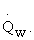This relationship and calculated data table shown by Haque 15 are provided below,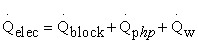(1)

Where,= V2/Relectrical = 36 W.

Equation (1) can be rewritten as,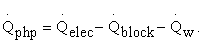(2)

Thus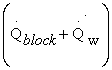gives the amount of total heat loss from the AC power supply to the working fluid.

Here,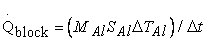(3)

Where, MAl= 7.5 kg, SAl= 900 J/kg˚C,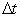= 20 min.

Conduction heat transfer through the PHP wall from the evaporator to the condenser section can be derived from the Fourier’s law of heat conduction,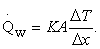(4)

Here, A= nπ(dO²-di²)/4, n= 14, ΔT=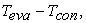Δx=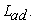Thermal conductivity of Aluminum, K=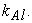Substituting all of these in the equation (4), we get,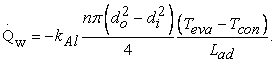(5)

In the current test set up, n = 14, do = 0.004 m, di = 0.003 m,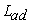= 0.12 m,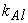=250 W/m °C

Overall Heat Transfer Coefficient,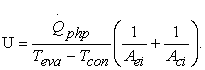(6)

### 4. Development of Empirical Correlation

From the analysis of experimental results of closed loop Pulsating Heat Pipe (CLPHP) discussed in this Chapter, the thermal performance of CLPHP depends on various parameters such as heat input, heat transfer coefficient, condenser inclination, evaporator temperature, ambient temperature and fill ratio.

4.1. Effect of Temperature Rise

A constant heat input of 36 W was applied in the heating block and temperature rise in various sections was monitored till steady state was reached. This is carried out for three different fill ratios, namely, 0.4, 0.6 and 0.8 and at 0°, 30°, 45°, 60°, 90° and 180° inclinations.

• Figure 3. Comparison of the rates of increase of temperature with time in the three sections of the CLPHP for fill ratio = 0.4 and inclination = 0° (Vertical position)
• Figure 4. Comparison of the rates of increase of temperature with time in the three sections of the CLPHP for fill ratio = 0.4 and inclination = 90° (Horizontal position)
• Figure 5. Comparison of the rates of increase of temperature with time in the three sections of the CLPHP for fill ratio = 0.4 and inclination = 180° (Heat source above the heat sink)

It is seen from the above figures that the difference in temperature between the evaporator and the condenser sections is uniform throughout the experimental range for 0° inclination. But for 90° and 180° inclinations this difference in temperature gradually increases with time. This observation again implies that the heat transfer rate decreases with increase of inclination above 90°.

• Figure 6. Comparison of the rates of increase of temperature with time in the three sections of the CLPHP for fill ratio = 0.6 and inclination = 0° (Vertical position)
• Figure 7. Comparison of the rates of increase of temperature with time in the three sections of the CLPHP for fill ratio = 0.6 and inclination = 90° (Horizontal position)
• Figure 8. Comparison of the rates of increase of temperature with time in the three sections of the CLPHP for fill ratio = 0.6 and inclination = 180° (Heat source above the heat sink)
• Figure 9. Comparison of the rates of increase of temperature with time in the three sections of the CLPHP for fill ratio = 0.8 and inclination = 0° (Vertical position)
• Figure 10. Comparison of the rates of increase of temperature with time in the three sections of the CLPHP for fill ratio = 0.8 and inclination = 90° (Horizontal position)
• Figure 11. Comparison of the rates of increase of temperature with time in the three sections of the CLPHP for fill ratio = 0.8 and inclination = 180° (Heat source above the heat sink).

It is seen from the above Figure 6 to Figure 11, that as with fill ratio =0.4, the difference in temperature between the evaporator and the condenser sections for the other two fill ratio is also uniform throughout the experimental range for 0° inclination. But for 90° and 180° inclinations this difference in temperature gradually increases with time though the rate of increase of temperature difference with 90o is very small. This observation again implies that the heat transfer rate decreases with increase of inclination above 90°.

• Figure 12. Effect of fill ratio(V/Vmax) on evaporator temperature T eva (°C) in CLPHP at different inclination.
• Figure 13. Effect of fill ratio(V/Vmax) on condenser temperature T con(°C) in CLPHP at different inclination.

It is seen from the above Figure 12 to Figure 13 that with fill ratio =0.4 and 0.6 for inclination angle below 90o the evaporator temperature is qualitatively and quantitavely same but for fill ratio 0.8 it is higher.For inclination angle 90° and above evaporator section temperature is much higher for all fill ratios.The condenser sections temperature for all fill ratios is lower for inclination angle 90° and 180°. This observation implies that the heat transfer rate is lower at fill ratio 0.8 compared to fill ratio 0.4 and 0.6. It also decreases with increase of inclination above 90°. It is also evident from Figure 12 that the temperature of evaporator section for fill ratios 0.4 and 0.6 is slightly lower in inclination angle 30°.

4.2. Effect of Heat Input

For fixed heat input 36W, heat transfer through the PHP has been calculated using equations from chapter 3.

• Figure 14. Effect of fill ratio(V/Vmax) on Heat transfer rate by working fluid , Qphp (Watt) at different inclination.

From Figure 14 above it is observed that for all fill ratios the Heat transfer rate, Qphp at inclinations 0°, 30°, 45°and 60° in are very close to each other though it is slightly lower for fill ratio 0.8 . It is also observed that for all fill ratios heat transfer rate, Qphp at inclinations 90°and 180° is much lower and it is lowest for fill ratio 0.8.So we can say that at fill ratios 0.4 and 0.6 heat transfer rate, Qphp is independent of inclination below 90°.

• Figure 15. Effect of inclination, θ on heat transfer rate by working fluid, Qphp (Watt) of CLPHP for different fill ratio at constant heat input

It is observed from Figure 15 that for all inclination below 90° heat transfer rate by working fluid, Qphp for fill ratio 0.4, 0.6 and 0.8 is approximately same though Qphp for 0.8 is slightly lower .For inclination 90° and 180° this Qphp is lower compared to other inclination. From fig it is also clear that Qphp for inclination 180° and fill ratio 0.8 is the lowest.

4.3. Empirical Correlation

In order to correlate the data obtained from the present experimental investigation, some dimensionless group such as heat input ratio, temperature difference ratio, ratio of condenser inclination and fill ratio are proposed. To determine the dependency of overall heat transfer ratio on the other dimensionless groups, the following steps are considered.

4.3.1. Heat Input Ratio

The present experimental investigations were carried out with different heat inputs. From the experimental results, it is found that, with the increase of heat input, the heat transfer rate is increased. For the analysis of heat transfer performance for different heat input, a ratio of heat input to the maximum heat input can be considered as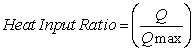Thus, the correlation can be assumed as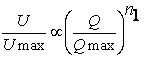(7)
• Figure 16. Determination of exponent ‘n1

In this case, using conventional techniques, the exponent n1 was determined by plotting (U/Umax) vs. (Q/Qmax) from the present experimental data as shown in Figure 16 and the average of the slope was found n1 = 0. 7545. So the correlation became: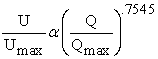(8)

4.3.2. Evaporator and Ambient Temperature

In the heat pipes, the heat is transferred by the evaporation and condensation of working fluid from evaporator and condenser section respectively. So, the heat transfer can be defined as the phase change heat transfer. Another mode of heat transfer can be occurred by the convection which is directly depends to the temperature differential of the evaporator and condenser section. From the experimental date, it is evident that, with the increase of temperature differential of evaporator to condenser section, the overall heat transfer coefficient also increases. From this, a dimensionless group can be assumed as following: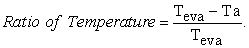This dimensionless parameter also should include the contribution of heat transfer performance of Looped Parallel Micro Heat Pipes (LPMHP). By including this dimensionless group the correlating equation became,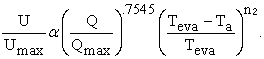(9)
• Figure 17. Determination of exponent ‘n2

In this case also using conventional techniques, the exponent n2 of the dimensionless heat transfer ratio was determined by plotting [U/Umax] / [(Q/Qmax)0.7545] vs. (Teva-Ta)/Teva) of the present experimental data as shown in Figure 17. The average value of the exponent was found to be, n2 = -.0575. So the Correlation became: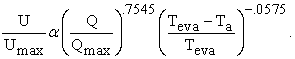(10)

4.3.3. Inclination of CLPHP

In the heat transfer performance of looped parallel micro heat pipe, the condenser inclination has an effect. For considering the effect of condenser inclination at the transport section, a dimensionless group can be assumed as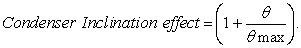Here, θ is the various condenser inclination and θmax is the maximum inclination angle which is at the horizontal position (θmax = 90o). By including this dimensionless group the correlating equation became,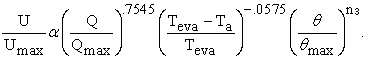(11)
• Figure 18. Determination of exponent ‘n3

In this case also using conventional techniques, exponent n3 of the group (1+θ/θmax) was determined by plotting [U/Umax] / (Q/Qmax)07545 X [(Teva-Ta)/Teva)].0575vs. (1+θ/θmax) of the present experimental data as shown in Figure 18. The average value of the exponent was found to be, n3 = 0.0777. So the final Correlation became: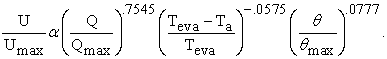(12)

4.3.4. CLPHP Fill Ratio

The fill ratio V/Vmax has an effect on the heat transfer performance CLPHP. For considering the effect of fill ratio at the transport section, a dimensionless group can be assumed as V/Vmax. Here, V is the various fill ratio and Vmax is the maximum fill ratio in the experiment. By including this dimensionless group, the correlating equation became,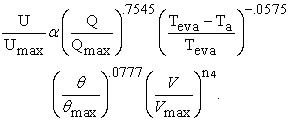(13)
• Figure 19. Determination of exponent ‘n4

In this case also using conventional techniques, exponent n4 of the group (V/Vmax) was determined by plotting (U/Umax)/[(Q/Qmax)07545X[(Teva-Ta)/Teva)].0575 X(1+θ/θmax).0777 vs (V/Vmax) of the present experimental data as shown in Figure 19 The average value of the exponent was found to be, n4 = 0.2021. So the final Correlation became: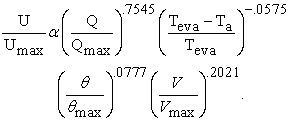(14)
• Figure 20. Graphical representation of correlation for CLPHP

4.3.5. Proposed Correlation

In this final correlation (equation 14), we found that the exponent of [(Teva-Ta)/Teva)^(-.0575)] and (1+θ/θmax) is very close to zero. In other words, it can be conclude that, the effect of [(Teva-Ta)/Teva)^(-.0575)] and (1+θ/θmax) dimensionless groups in the heat transfer characteristic of CLPHP is very insignificant. In this case, if we neglect these two terms, thus the correlation becomes: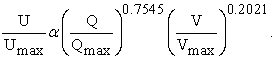(15)
• Figure 21. Proposed Correlation

Then the graphical representation of the simplified correlations for LPMHP is shown in Figure 21. The complete correlation for pulsating heat pipe is given by the following equation: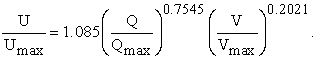(16)

### 5. Conclusion

This paper aims at building up an empirical equation considering the physical parameters that directly contributes to heat transfer through the pulsating heat pipe. Heat input, Evaporator temperature rise, inclination and fill ratios are compared to form a linear empirical relationship. From the section of 4.1 and 4.2 it is recommended that the best performance can be achieved at inclination below 90˚ and fill ratios 0.4 and 0.6. It is found that overall heat transfer coefficient varies effectively with the heat input to the PHP and the fill ratios. It is evident that the final correlation converges well for linear relationship.

### Acknowledgements

This work is supported by Bangladesh Army, Department of Mechanical Engineering, Bangladesh Military Academy, Bhatiary, Chittagong, Bangladesh.

### Nomenclature

A: Cross sectional area of the wall, m2

Aci: Inner surface area of the condenser, m2

Aei: Inner surface area of the evaporator, m2

do: Outer diameter of the Aluminum pipe, m

di: Inner diameter of the Aluminum pipe, m

h: Convective heat transfer coefficient ,W/m2 °C

K: Thermal conductivity of metal, W/m.K

KAl: Thermal conductivity of Aluminum, W/m.K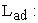Length of the PHP wall between the evaporator and the condenser, m

MAl: Mass of Aluminum block, Kg

n: Number of turns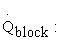Heat absorbed by the block, W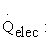Electric heat input, W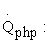Rate of Heat transfer by the working fluid, W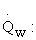Heat conduction through the pipe wall, W

SAl: Specific heat of Aluminum, J/Kg°C

Ta: Ambient temperature, °C

Tcon: Average temperature of conduction section, °C

Teva: Average temperature of evaporator section, °C

TAl: Rise of temperatute of Aluminum block at the time interval Δt, °C

ΔT: Temperature difference between evaporator and condenser, °C

Δt: Time interval, sec

U: Overall heat transfer coefficient, W/m2. °C

V: Fill Ratio.

### References

  N. Tesla, "Valvular Conduit". New York Patent US 1329559 A, 3 February 1920. In article  R. S. Gaugler, "HEAT TRANSFER DEVICE". Dayton, Ohio Patent 2,350,348, 6 June 1944. In article  H. Akachi, "STRUCTURE OF MICRO-HEAT PIPE". Sagamihara, Japan Patent 5,219,020, 15 August 1991. In article  G. M. Grover, "Evaporation-Condenssation Heat Transfer Device". US Patent 3229759, 2 December 1963. In article  P. a. R. Dunn, Heat Pipes, Pergamon Press, 1982. In article  A. Faghri, "Review and Advances in Heat Pipe Science and Technology," Journal of heat Transfer, vol. 134, no. 12, p. 18 pages, 2012. In article View Article  X. J. L. a. Z. Z. Q. Zhang X. M., "Experimental Study of a Pulsating Heat pipe using FC-72, Ethanol and Water as working fluid," vol. 12, pp. 445-472, 1999. In article  B. a. F. Holley, "Analysis of Pulsating Heat Pipe with Capillary Wick and Varying Channel Diameter," International Journal of Heat and Mass Transfer , vol. 48, pp. 2635-2651, 2005. In article View Article  T. Dobson R.T. and Harms, "Lumped Parameter Analysis of Closed and Open Oscillatory Heat Pipes," in 11th International Heat Pipe Conference , Tokyo, Japan , 1999. In article  S. K. M. G. Honghai Yang, "Performance characteristics of pulsating heat pipes as integral thermal spreaders," International Journal of Thermal Sciences, vol. 48, pp. 815-824, 2009. In article View Article  Khandekar S. and Groll M., "An Insight into Thermo-Hydraulic Coupling in Pulsating Heat Pipes," Int. J. of Thermal Sciences, vol. 43, no. 1, pp. 13-20, 2004. In article View Article  B. Y. W. T. N. a. O. K. T. Tong, " Closed-loop pulsating heat pipe," Applied Thermal Engineering, vol. 21, p. 1845-1862, 2001. In article View Article  A. F. Y. Z. M.B.Shafi, " Thermal modeling of unlopped and looped pulsating heat pipes," ASME J. Heat Transfer , vol. 123, pp. 1159-1172, 2001. In article View Article  P. T. T. W. P. K. M. M. P Sakulchangsatjatai, "Operation modeling of closed end and closed loop oscillating heat pipe at normal operat-ing condition," Appl. Therm. Eng., vol. 24, pp. 995-1008, 2004. In article View Article  A. M. M. F. K. a. M. M. R. Md Shahidul Haque, "Thermal characteristics of an ammonia-charged closed-loop pulsating heat pipe," Journal of Mechanical Science and Technology, vol. 33, no. 4, pp. 1907-1914, 2019. In article View Article

Published with license by Science and Education Publishing, Copyright © 2020 Md Shahidul Haque, Antara Majumdar, Md. Faisal Kader and Md. Mahbubur RazzaqThis work is licensed under a Creative Commons Attribution 4.0 International License. To view a copy of this license, visit http://creativecommons.org/licenses/by/4.0/